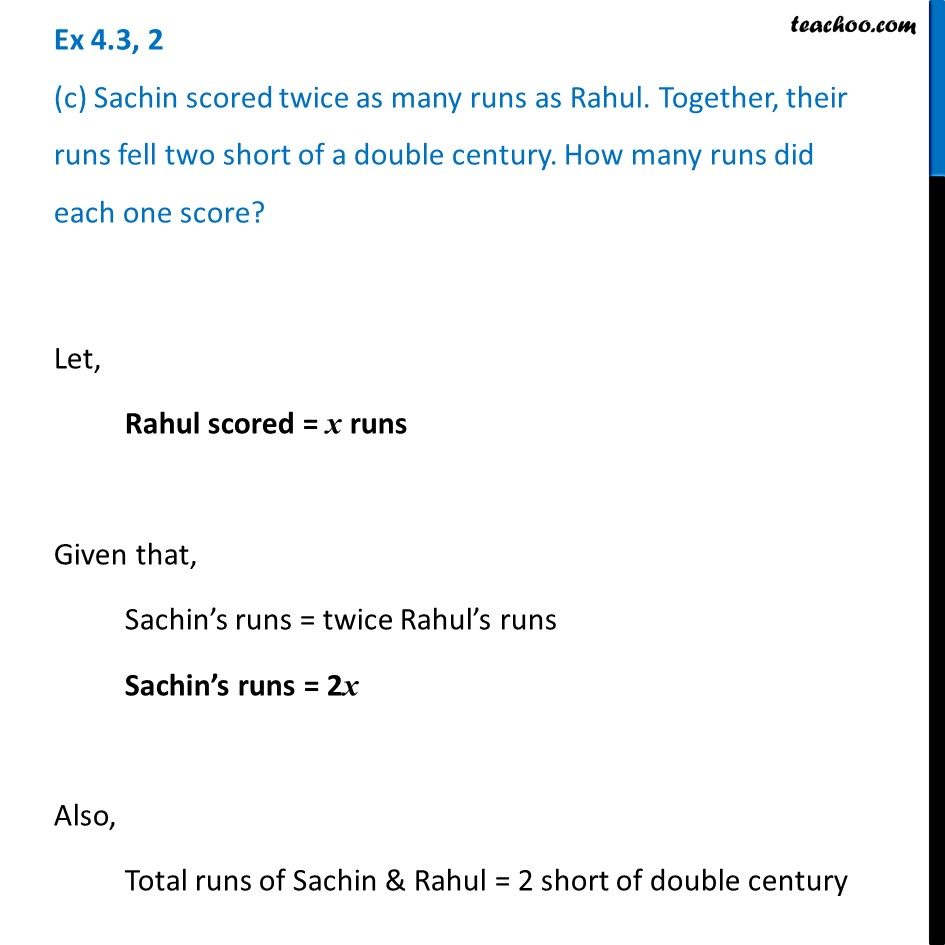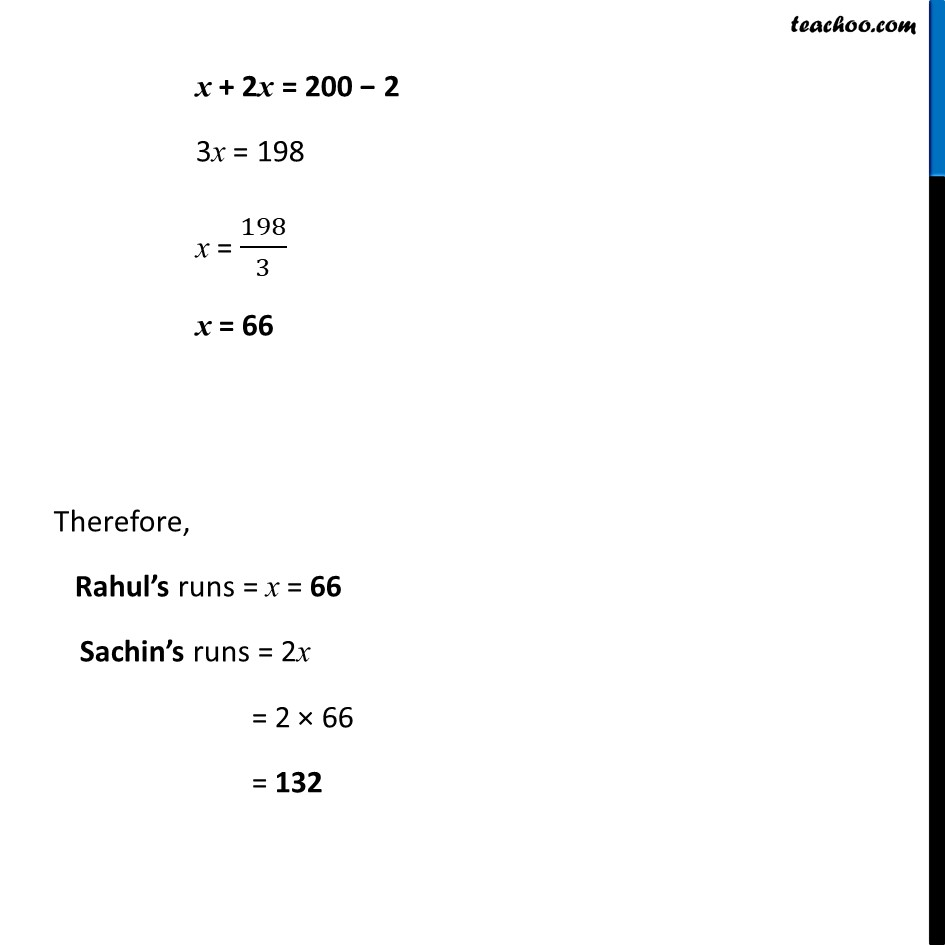Ex 4.3

Chapter 4 Class 7 Simple Equations
Serial order wiseLearn in your speed, with individual attention - Teachoo Maths 1-on-1 Class

### Transcript

Ex 4.3, 2 (c) Sachin scored twice as many runs as Rahul. Together, their runs fell two short of a double century. How many runs did each one score?Let, Rahul scored = x runs Given that, Sachin’s runs = twice Rahul’s runs Sachin’s runs = 2x Also, Total runs of Sachin & Rahul = 2 short of double century x + 2x = 200 − 2 3x = 198 x = 198/3 x = 66 Therefore, Rahul’s runs = x = 66 Sachin’s runs = 2x = 2 × 66 = 132